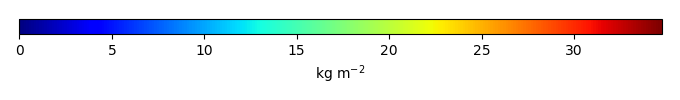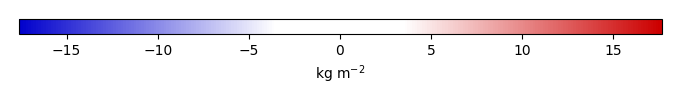# Mean State

Period Mean (original grids) [Pg]
Model Period Mean (intersection) [Pg]
Model Period Mean (complement) [Pg]
Benchmark Period Mean (intersection) [Pg]
Benchmark Period Mean (complement) [Pg]
Bias [kg m-2]
Bias Score 
Spatial Distribution Score 
Overall Score 
Benchmark [-] 64.0
CLM5PHSOFF [-] 47.0 46.0 1.24 63.9 0.0663 -5.30 0.69 0.77 0.73
CLM5PHSON [-] 38.4 38.4 0.429 63.9 0.0663 -7.15 0.63 0.58 0.61
Period Mean (original grids) [Pg]
Model Period Mean (intersection) [Pg]
Model Period Mean (complement) [Pg]
Benchmark Period Mean (intersection) [Pg]
Benchmark Period Mean (complement) [Pg]
Bias [kg m-2]
Bias Score 
Spatial Distribution Score 
Overall Score 
Benchmark [-] 3.34
CLM5PHSOFF [-] 2.39 1.45 0.643 3.34 0.00700 -0.601 0.48 0.55 0.51
CLM5PHSON [-] 3.30 2.21 0.738 3.34 0.00700 -0.331 0.50 0.57 0.53
Period Mean (original grids) [Pg]
Model Period Mean (intersection) [Pg]
Model Period Mean (complement) [Pg]
Benchmark Period Mean (intersection) [Pg]
Benchmark Period Mean (complement) [Pg]
Bias [kg m-2]
Bias Score 
Spatial Distribution Score 
Overall Score 
Benchmark [-] 14.1
CLM5PHSOFF [-] 10.4 8.13 1.85 14.1 0.0194 -0.876 0.48 0.75 0.62
CLM5PHSON [-] 12.3 11.1 0.996 14.1 0.0194 -0.223 0.55 0.79 0.67
Period Mean (original grids) [Pg]
Model Period Mean (intersection) [Pg]
Model Period Mean (complement) [Pg]
Benchmark Period Mean (intersection) [Pg]
Benchmark Period Mean (complement) [Pg]
Bias [kg m-2]
Bias Score 
Spatial Distribution Score 
Overall Score 
Benchmark [-] 1.22
CLM5PHSOFF [-] 3.49 2.66 0.855 1.22 0.00453 0.572 0.51 0.68 0.60
CLM5PHSON [-] 4.18 3.37 0.817 1.22 0.00453 0.991 0.47 0.50 0.49
Period Mean (original grids) [Pg]
Model Period Mean (intersection) [Pg]
Model Period Mean (complement) [Pg]
Benchmark Period Mean (intersection) [Pg]
Benchmark Period Mean (complement) [Pg]
Bias [kg m-2]
Bias Score 
Spatial Distribution Score 
Overall Score 
Benchmark [-] 47.3
CLM5PHSOFF [-] 78.7 67.4 9.09 45.9 1.37 6.09 0.71 0.70 0.70
CLM5PHSON [-] 40.1 38.1 1.81 45.9 1.37 2.33 0.68 0.51 0.60
Period Mean (original grids) [Pg]
Model Period Mean (intersection) [Pg]
Model Period Mean (complement) [Pg]
Benchmark Period Mean (intersection) [Pg]
Benchmark Period Mean (complement) [Pg]
Bias [kg m-2]
Bias Score 
Spatial Distribution Score 
Overall Score 
Benchmark [-] 41.6
CLM5PHSOFF [-] 32.6 31.5 0.606 41.6 0.0303 -1.13 0.51 0.71 0.61
CLM5PHSON [-] 52.3 51.1 0.450 41.6 0.0303 1.23 0.55 0.74 0.65
Period Mean (original grids) [Pg]
Model Period Mean (intersection) [Pg]
Model Period Mean (complement) [Pg]
Benchmark Period Mean (intersection) [Pg]
Benchmark Period Mean (complement) [Pg]
Bias [kg m-2]
Bias Score 
Spatial Distribution Score 
Overall Score 
Benchmark [-] 15.6
CLM5PHSOFF [-] 27.8 23.5 4.20 15.6 0.0349 0.837 0.48 0.66 0.57
CLM5PHSON [-] 31.8 29.6 2.96 15.6 0.0349 1.93 0.51 0.68 0.59
Period Mean (original grids) [Pg]
Model Period Mean (intersection) [Pg]
Model Period Mean (complement) [Pg]
Benchmark Period Mean (intersection) [Pg]
Benchmark Period Mean (complement) [Pg]
Bias [kg m-2]
Bias Score 
Spatial Distribution Score 
Overall Score 
Benchmark [-] 14.8
CLM5PHSOFF [-] 33.0 27.3 3.92 14.6 0.136 2.09 0.48 0.63 0.56
CLM5PHSON [-] 24.9 22.6 1.43 14.6 0.136 2.37 0.53 0.73 0.63
Period Mean (original grids) [Pg]
Model Period Mean (intersection) [Pg]
Model Period Mean (complement) [Pg]
Benchmark Period Mean (intersection) [Pg]
Benchmark Period Mean (complement) [Pg]
Bias [kg m-2]
Bias Score 
Spatial Distribution Score 
Overall Score 
Benchmark [-] 1.78
CLM5PHSOFF [-] 5.64 4.17 1.82 1.78 0.00 1.19 0.48 0.67 0.57
CLM5PHSON [-] 6.90 5.31 1.98 1.78 0.00 1.79 0.40 0.58 0.49
Period Mean (original grids) [Pg]
Model Period Mean (intersection) [Pg]
Model Period Mean (complement) [Pg]
Benchmark Period Mean (intersection) [Pg]
Benchmark Period Mean (complement) [Pg]
Bias [kg m-2]
Bias Score 
Spatial Distribution Score 
Overall Score 
Benchmark [-] 15.3
CLM5PHSOFF [-] 18.5 13.5 4.37 14.8 0.505 -0.314 0.53 0.81 0.67
CLM5PHSON [-] 11.3 9.25 1.86 14.8 0.505 -0.174 0.55 0.81 0.68
Period Mean (original grids) [Pg]
Model Period Mean (intersection) [Pg]
Model Period Mean (complement) [Pg]
Benchmark Period Mean (intersection) [Pg]
Benchmark Period Mean (complement) [Pg]
Bias [kg m-2]
Bias Score 
Spatial Distribution Score 
Overall Score 
Benchmark [-] 13.1
CLM5PHSOFF [-] 21.3 17.6 3.17 13.1 0.0236 0.832 0.46 0.68 0.57
CLM5PHSON [-] 18.2 17.0 0.899 13.1 0.0236 1.50 0.50 0.69 0.60
Period Mean (original grids) [Pg]
Model Period Mean (intersection) [Pg]
Model Period Mean (complement) [Pg]
Benchmark Period Mean (intersection) [Pg]
Benchmark Period Mean (complement) [Pg]
Bias [kg m-2]
Bias Score 
Spatial Distribution Score 
Overall Score 
Benchmark [-] 455.
CLM5PHSOFF [-] 543. 484. 59.8 449. 5.38 0.329 0.64 0.89 0.76
CLM5PHSON [-] 487. 464. 23.3 449. 5.38 0.776 0.64 0.86 0.75
Period Mean (original grids) [Pg]
Model Period Mean (intersection) [Pg]
Model Period Mean (complement) [Pg]
Benchmark Period Mean (intersection) [Pg]
Benchmark Period Mean (complement) [Pg]
Bias [kg m-2]
Bias Score 
Spatial Distribution Score 
Overall Score 
Benchmark [-] 0.854
CLM5PHSOFF [-] 0.970 0.441 0.430 0.849 0.00531 -0.259 0.42 0.66 0.54
CLM5PHSON [-] 1.51 0.664 0.856 0.849 0.00531 0.0462 0.48 0.72 0.60
Period Mean (original grids) [Pg]
Model Period Mean (intersection) [Pg]
Model Period Mean (complement) [Pg]
Benchmark Period Mean (intersection) [Pg]
Benchmark Period Mean (complement) [Pg]
Bias [kg m-2]
Bias Score 
Spatial Distribution Score 
Overall Score 
Benchmark [-] 2.82
CLM5PHSOFF [-] 11.6 9.02 2.37 2.82 0.00299 1.89 0.37 0.35 0.36
CLM5PHSON [-] 14.5 12.3 2.08 2.82 0.00299 3.01 0.27 0.35 0.31
Period Mean (original grids) [Pg]
Model Period Mean (intersection) [Pg]
Model Period Mean (complement) [Pg]
Benchmark Period Mean (intersection) [Pg]
Benchmark Period Mean (complement) [Pg]
Bias [kg m-2]
Bias Score 
Spatial Distribution Score 
Overall Score 
Benchmark [-] 4.95
CLM5PHSOFF [-] 16.3 13.3 2.28 4.88 0.0615 3.12 0.38 0.40 0.39
CLM5PHSON [-] 10.2 9.16 0.789 4.88 0.0615 3.11 0.45 0.52 0.48
Period Mean (original grids) [Pg]
Model Period Mean (intersection) [Pg]
Model Period Mean (complement) [Pg]
Benchmark Period Mean (intersection) [Pg]
Benchmark Period Mean (complement) [Pg]
Bias [kg m-2]
Bias Score 
Spatial Distribution Score 
Overall Score 
Benchmark [-] 13.0
CLM5PHSOFF [-] 7.07 5.61 1.10 13.0 0.00854 -1.84 0.48 0.63 0.55
CLM5PHSON [-] 11.8 11.4 0.332 13.0 0.00854 -0.100 0.65 0.87 0.76
Period Mean (original grids) [Pg]
Model Period Mean (intersection) [Pg]
Model Period Mean (complement) [Pg]
Benchmark Period Mean (intersection) [Pg]
Benchmark Period Mean (complement) [Pg]
Bias [kg m-2]
Bias Score 
Spatial Distribution Score 
Overall Score 
Benchmark [-] 21.4
CLM5PHSOFF [-] 32.7 31.6 1.13 21.3 0.128 1.87 0.48 0.69 0.58
CLM5PHSON [-] 43.8 43.5 0.471 21.3 0.128 4.72 0.47 0.79 0.63
Period Mean (original grids) [Pg]
Model Period Mean (intersection) [Pg]
Model Period Mean (complement) [Pg]
Benchmark Period Mean (intersection) [Pg]
Benchmark Period Mean (complement) [Pg]
Bias [kg m-2]
Bias Score 
Spatial Distribution Score 
Overall Score 
Benchmark [-] 9.57
CLM5PHSOFF [-] 1.47 1.24 0.324 9.53 0.0397 -2.59 0.40 0.26 0.33
CLM5PHSON [-] 3.09 2.90 0.233 9.53 0.0397 -1.92 0.49 0.49 0.49
Period Mean (original grids) [Pg]
Model Period Mean (intersection) [Pg]
Model Period Mean (complement) [Pg]
Benchmark Period Mean (intersection) [Pg]
Benchmark Period Mean (complement) [Pg]
Bias [kg m-2]
Bias Score 
Spatial Distribution Score 
Overall Score 
Benchmark [-] 151.
CLM5PHSOFF [-] 150. 147. 2.37 151. 0.0403 -0.581 0.78 0.93 0.86
CLM5PHSON [-] 129. 128. 0.570 151. 0.0403 -2.41 0.76 0.78 0.77
Period Mean (original grids) [Pg]
Model Period Mean (intersection) [Pg]
Model Period Mean (complement) [Pg]
Benchmark Period Mean (intersection) [Pg]
Benchmark Period Mean (complement) [Pg]
Bias [kg m-2]
Bias Score 
Spatial Distribution Score 
Overall Score 
Benchmark [-] 10.7
CLM5PHSOFF [-] 20.3 19.1 1.41 10.7 0.0170 1.19 0.51 0.78 0.64
CLM5PHSON [-] 21.5 21.4 0.512 10.7 0.0170 1.83 0.52 0.81 0.66
Period Mean (original grids) [Pg]
Model Period Mean (intersection) [Pg]
Model Period Mean (complement) [Pg]
Benchmark Period Mean (intersection) [Pg]
Benchmark Period Mean (complement) [Pg]
Bias [kg m-2]
Bias Score 
Spatial Distribution Score 
Overall Score 
Benchmark [-] 4.41
CLM5PHSOFF [-] 8.10 7.47 0.837 4.31 0.102 1.33 0.56 0.76 0.66
CLM5PHSON [-] 6.25 5.78 0.493 4.31 0.102 1.41 0.59 0.80 0.70

# Temporally integrated period mean

BENCHMARK MEANMODEL MEANBIASBIAS SCORESPATIAL TAYLOR DIAGRAMMODEL COLORS# Spatially integrated regional mean

MODEL COLORS# All Models

BenchmarkCLM5PHSOFFCLM5PHSON# Data Information

history: Mon Mar 21 09:05:59 2016: ncatted -a units,biomass,o,c,kg m-2 ./from_MQs_site/DATA/biomass/GEOCARBON/derived/biomass_0.5x0.5.nc tmp.nc